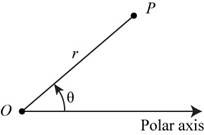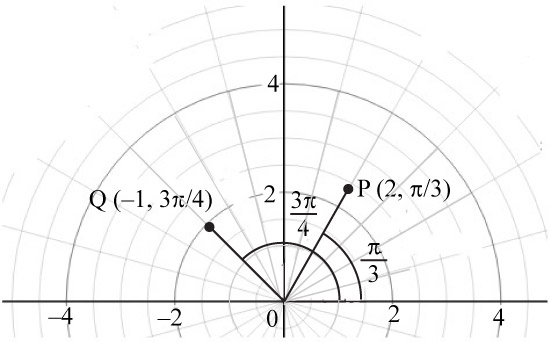# (a) Explain the polar coordinate system. (b) Graph the points with polar coordinates (2, π /3) and (−1, 3 π /4). (c) State the equations that relate the rectangular coordinates of a point to its polar coordinates. (d) Find rectangular coordinates for (2, π /3). (e) Find polar coordinates for P (−2, 2).### Precalculus: Mathematics for Calcu...

7th Edition
James Stewart + 2 others
Publisher: Brooks Cole
ISBN: 9781305071759### Precalculus: Mathematics for Calcu...

7th Edition
James Stewart + 2 others
Publisher: Brooks Cole
ISBN: 9781305071759

#### Solutions

Chapter
Section
Chapter 8, Problem 1RCC
Textbook Problem

## (a) Explain the polar coordinate system. (b) Graph the points with polar coordinates (2, π/3) and (−1, 3π/4). (c) State the equations that relate the rectangular coordinates of a point to its polar coordinates. (d) Find rectangular coordinates for (2, π/3). (e) Find polar coordinates for P(−2, 2).

Expert Solution

(a)

To determine

To describe: The polar coordinate system.

### Explanation of Solution

The coordinate system (r,θ) signifies the polar coordinates with r as radius and θ as the angle between the polar axis and the line segment joining the point.

In polar coordinate system r shows the distance of the point and θ shows the direction of the polar coordinate (r,θ) .

In polar coordinate system take θ positive in counter clockwise direction else take θ negative in clockwise direction.

In polar coordinate system negative r signifies that the polar coordinate (r,θ) lies |r| units from the pole in opposite direction of angle θ .

The below figure shows the polar coordinates (r,θ) .Figure (1)

In the above figure, the point P is r unit away adjoining with angle θ .

Expert Solution

(b)

To determine

To sketch: The graph of the polar coordinates.

### Explanation of Solution

The below graph shows the polar coordinates (2,π3) and (1,3π4) .Figure (2)

In the above graph, point P(2,π3) and Q(1,3π4) show the required points in polar coordinate system.

Expert Solution

(c)

To determine

To describe: The equations that relate the rectangular coordinates and polar coordinates of a point with each other.

### Explanation of Solution

Use the equations x=rcosθ and y=rsinθ to convert the polar coordinate system of an equation to its corresponding rectangular coordinate system.

Use the equations r2=x2+y2 and tanθ=yx(x0) to convert rectangular coordinate system of a point to its corresponding polar coordinate system.

Expert Solution

(d)

To determine

To find: The rectangular coordinate of the point.

The rectangular coordinate of the point (2,π3) is (1,3) .

### Explanation of Solution

Given:

The value of polar coordinate is (2,π3) .

Calculation:

Use the equations x=rcosθ and y=rsinθ to convert the polar coordinate system of a equation to its corresponding rectangular coordinate system.

The formula to calculate the x coordinate is,

x=rcosθ .

Substitute 2 for r and π3 for θ in the above formula.

x=2cosπ3=2×12=1

The value of the x coordinate is 1.

The formula to calculate the y coordinate is,

y=rsinθ .

Substitute 2 for r and π3 for θ in the above formula.

y=2sinπ3=2×32=3

The value of the y coordinate is 3 .

Thus, the rectangular coordinate of the point (2,π3) is (1,3) .

Expert Solution

(e)

To determine

To find: The polar coordinate of the point.

The polar coordinate of the point (2,2) is (22,π4) .

### Explanation of Solution

Given:

The value of rectangular coordinate is (2,2) .

Calculation:

Use the equations r2=x2+y2 and tanθ=yx(x0) to convert rectangular coordinate system of a point to its corresponding polar coordinate system.

The formula to calculate the r is,

r2=x2+y2

Substitute 2  for x and 2 for y in the above formula.

r2=(2)2+(2)2=4+4=8=22

The value of r is 22 .

The formula to calculate the value of θ is,

tanθ=yx(x0)

Substitute 2 for x and 2 for y in the above formula,

tanθ=22=1tan1(tanθ)=tan1(1)(Taketan1θboth sides)θ=π4(tan1(tanθ)=θ)

The value of θ is π4 .

Thus, the rectangular coordinate of the point (2,2) is (22,π4) .

### Want to see more full solutions like this?

Subscribe now to access step-by-step solutions to millions of textbook problems written by subject matter experts!

Get Solutions

### Want to see more full solutions like this?

Subscribe now to access step-by-step solutions to millions of textbook problems written by subject matter experts!

Get Solutions